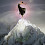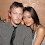## Sunday, January 31, 2016

### 38 41 50 68 83 86 119 144 223 227 | The Death of Terry Wogan of BBC, January 31, 2016, 7-Days before Super Bowl 50

Sir = 1+9+9 = 19/28 (Man = 28)
Michael = 4+9+3+8+1+5+3 = 33
Terence = 2+5+9+5+5+3+5 = 34
Terry = 2+5+9+9+7 = 32
Wogan = 5+6+7+1+5 = 24
Sir Michael Terrence Wogan = 110/119 (All seeing eye) (Star of David)
Terry Wogan = 56 (All seeing eye) (Star of David)

Sir = 19+9+18 = 46
Michael = 13+9+3+8+1+5+12 = 51
Terence = 20+5+18+5+14+3+5 = 70
Terry = 20+5+18+18+25 = 86
Wogan = 23+15+7+1+14 = 60
Sir Michael Terrence Wogan = 227
Terry Wogan = 146

How about that gematria?  Keep in mind this guy worked for the 'BBC', or 'The Synagogue of Satan'.

BBC = 223

8/3/1938 = 8+3+19+38 = 68 (San Francisco = 68)
8/3/1938 = 8+3+1+9+3+8 = 32 (UK) (America) (7/4/1776) (NFL)
8/3/38 = 8+3+38 = 49 (7x7 = 49) (49ers hosting Super Bowl 50)

Notice he was born on August 3, written 8/3 or 3/8.  There are a number of synchronicities connecting his death to the upcoming Super Bowl.  Each time a major sports championship approaches, celebrities often die in ritualistic fashion, in relation to the big event.

Football = 6+15+15+20+2+1+12+12 = 83
The Super Bowl is on the 38th day of the year

1/31/2016 = 1+31+20+16 = 68
1/31/2016 = 1+31+(2+0+1+6) = 41 (Super Bowl) (Broncos)
1/31/2016 = 1+3+1+2+0+1+6 = 14 (Dead)
1/31/16 = 1+31+16 = 48

1/31....
Super Bowl = 19+21+16+5+18+2+15+23+12 = 131
Championship = 3+8+1+13+16+9+15+14+19+8+9+16 = 131

Notice that Terry was born on a date with '68' numerology and died on a date with '68' numerology.  The numbers 68, 41, and 14 all have numerology of 5, as does '77', his age of death.  The Broncos first made the NFL playoffs in '77.

Broncos = 2+9+6+5+3+6+1 =  32/41
Denver = 4+5+5+4+5+9 = 32
NFL = 14+6+12 = 32
America = 1+4+5+9+9+3+1 = 32
UK = 21+11 = 32
7/4/1776 = 7+4+1+7+7+6 = 32

What stands out to me, is how well his number synch up with the Denver Broncos and American football, with his death coming a week before the Super Bowl that the Broncos are playing in.  But why sacrifice a man from the UK?  Perhaps because they're a nation flirting with acquiring an NFL football team...

The Super Bowl is also in San Francisco this year... #50, and Terry's career with the BBC lasted 50-years.

San Francisco = 50/59/68
Super Bowl = 1+3+7+5+9+2+6+5+3 = 41/50

Tony Hall, eh?  BBC director, eh?  This might be the guy who gave him the fatal medicine.

Tony = 20+15+14+25 = 74 (Masonic = 74) (London = 74)
Hall = 8+1+12+12 = 33
Tony Hall = 107 (Kill = 2+9+3+3 = 17)

That 50-year career cannot be overlooked.  That is long time to work for the gang, a gang that shows no loyalty to no man.

Robert, Hall?  33, 33, 33... what else could we expect from the BBC?  "Lord Hall"... good god.

Lord = 12+15+18+4 = 49

1972-1984, 12-years.  Recall, 12 represents complete in numerology.

Then form 1993 to 2009, a span of sixteen years, that number with the masonic '33', '42' and '96' connection.

Sixteen = 1+9+6+2+5+5+5 = 33/42
Sixteen = 19+9+24+20+5+5+14 = 96

The richest families I knew growing up, the Norton family and the Chambers family.

Norton = 5+6+9+2+6+5 = 33
Chambers = 3+8+1+4+2+5+9+1 = 33/42

Of course PM '33' weighed in.

Cameron = = 3+1+4+5+9+6+5 = 33

That final air date is a can't miss detail.

11/8/2015 = 11+8+20+15 = 54 (Number attached to sacrifice)
11/8/2015 = 11+8+(2+0+1+5) = 27 (27 Club)
11/8/2015 = 1+1+8+2+0+1+5 = 18 (6+6+6)
11/8/15 = 11+8+15 = 34 (Murder = 34)

And let us not overlook the 118 sequence from November 8.
http://www.bbc.com/news/entertainment-arts-35453541

Recently, on September 10, 2015, Wogan said he would remain working for another 10-years.

His death comes 144-days after his announcement that he would work for another ten years.  144 is a number connected to murder, as well as time.

1.Nice work Zach.
A lot if people on fb saying what a great Irish person he was.
Fuck him!! He sold out his Irishness.

1.Yea, if you work for the BBC for 50-years, you're in the gang.

2.The 33 Gang must be destroyed.

2.You do not disappoint! I wouldn't have thought you would have any inkling of Sir Terry...well done :-) Interesting links....

3.Hey Zac,
4.